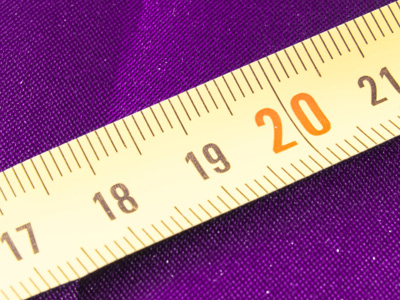We use mm to measure the length or height of small things, cm for larger things, metres for very large things and km for long distances.

# Year 2 Measurements - Estimating

This quiz addresses the requirements of the National Curriculum KS1 Maths and Numeracy for children aged 6 and 7 in year 2. Specifically this quiz is aimed at the section dealing with choosing and using appropriate standard units to estimate and measure length/height in any direction (m/cm), mass (kg/g), temperature (°C) and capacity (litres/ml) to the nearest appropriate unit.

Estimating measurements means being able to make a sensible guess as to a length, height, weight, temperature or capacity and choose the appropriate unit to do so. This quiz will help your child to choosing the appropriate units to measure different properties.

To see a larger image, click on the picture.
Question 1
How much liquid could a coffee cup hold?
250g
250cm
250ml
250kg
Millilitres are used to measure smaller amounts of liquid
Question 2
What unit of measurement would be most appropriate to measure the length of this pitch?
Degrees
Kilograms
Millilitres
Metres
Metres would be easier than centimetres!
Question 3
How much might this puppy weigh?
5oC
5g
5km
5kg
Kilograms would be the best unit to use here
Question 4
What unit of measure would be best to measure the ribbon around the parcel?
Metres
Centimetres
Millimetres
Miles
Centimetres would be easier to use for shorter measurements
Question 5
What could the temperature be in this fridge?
2mm
2oC
2kg
2m
Temperatures are measured in degrees
Question 6
How tall might this man be?
1m 80cm
18cm
1km 8m
12m
Most adults are taller than 1 metre but less than 2 metres
Question 7
What could be the temperature in this photograph?
80mm
80ml
80km
80oC
Degrees measure temperatures. 80oC is very hot!
Question 8
How long could your hand be?
12cm
12mm
12m
12km
Smaller items are usually measured in centimetres
Question 9
How much lemonade might this jug hold?
2 metres
2 litres
2 millimetres
2 kilograms
Litres are used for liquid
Question 10
What could this item be used to measure?
A large bunch of bananas
The water in a bath
The distance to the moon
The height of a cat
These are kitchen scales and are usually used to weigh items of food
Author:  Angela Smith